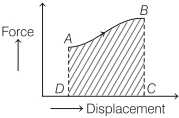# What is Work in Physics | Definition, Formula, Units – Work, Energy and Power

Work Physics Definition:
When a force acts on an object such that it displaces through some distance in the direction of applied force, then the work is said to be done by the force.

We are giving a detailed and clear sheet on all Physics Notes that are very useful to understand the Basic Physics Concepts.

## What is Work in Physics | Definition, Formula, Units – Work, Energy, and Power

Work done by the force is equal to the product of the force and the displacement of the object in the direction of force.

If under a constant force F the object is displaced through a distance s, then work done by the force

W = Fs = Fs cos θ

where, θ is the smaller angle between F and s.
Work is a scalar quantity.

Work Units in Physics:
Its SI unit is joule and CGS unit is erg.

∴ 1 joule = 107 erg

Dimensional Formula of Work:
Its dimensional formula is [ML2T-2].

Work done by a force is zero, if
(a) body is not displaced actually, i.e. s = 0.
(b) body is displaced perpendicular to the direction of force, i.e. θ = 90°.

• Work done by a force is positive, if angle between F and s is acute angle.
• Work done by a force is negative, if angle between F and s is obtuse angle.
• Work done by a constant force depends only on the initial and final positions of the object and not on the actual path followed between initial and final positions.

Work done in different conditions
(i) Work done by a variable force is given by

$$W=\int \mathbf{F} \cdot \mathbf{d s}$$

It is equal to the area under the force-displacement graph, along with proper sign.Work done = Area ABCDA

(ii) Work done in displacing any body under the action of a number of forces is equal to the work done by the resultant force.

(iii) In equilibrium (static or dynamic), the resultant force is zero, therefore resultant work done is zero.

(iv) If work done by a force during a rough trip of a system is zero, then the force is conservative, otherwise it is called non-conservative force.

• Gravitational force, electrostatic force, magnetic force etc are conservative forces. All the central forces are conservative forces.
• Frictional force, viscous force etc are non-conservative forces.

(v) Work done by the force of gravity on a particle of mass m is given by W = mgh
where, g is acceleration due to gravity and h is height through which the particle is displaced.

(vi) Work done in compressing or stretching a spring is given by

W = $$-\frac{1}{2}$$kx²

where, k is spring constant and x is displacement from mean position.

(vii) When on end of a spring is attached to a fixed vertical support and a block attached to the free end moves on a horizontal
table from x = x1 to x = x2, then W = $$\frac{1}{2}$$ k (x²2 – x1²).

(viii) Work done by the couple for an angular displacement θ is given by W = τ • θ, where τ is the torque of the couple.

Work, Energy and Power:
Work, energy and power are the three quantities which are inter-related to each other. The rate of doing work is called power. An equal amount of energy is consumed to do a work. So, basically the power is the rate at which energy is consumed to complete a work.